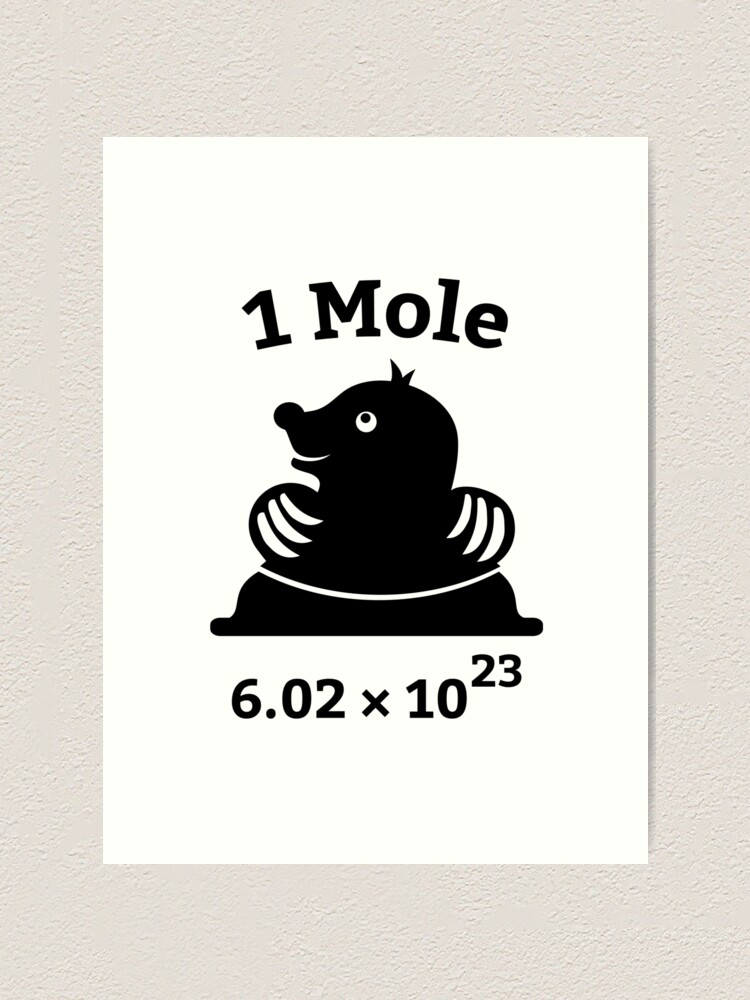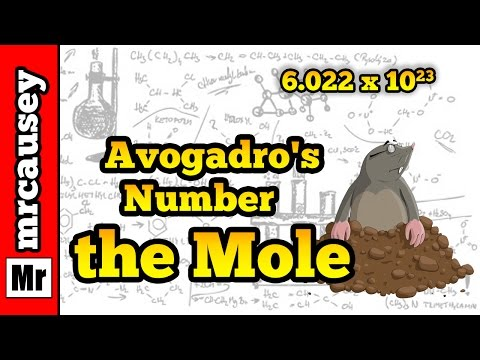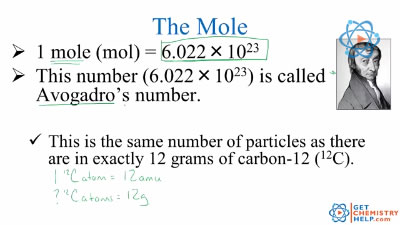The chemical changes observed in any reaction involve the rearrangement of billions of atoms. It is impractical to try to count or visualize all these atoms, but scientists need some way to refer to the entire quantity. They also need a way to compare these numbers and relate them to the weights of the substances, which they can measure and observe. The solution is the concept of the mole, which is very important in quantitative chemistry.Amadeo Avogadro first proposed that the volume of a gas at a given pressure and temperature is proportional to the number of atoms or molecules, regardless of the type of gas. Although he did not determine the exact proportion, he is credited for the idea.

A mole is a quantity that contains 6.02 *1023 atoms, molecules and ions.

Avogadro’s number is the number of particles in a mole 6.02 *1023

The mole (or mol) represents a certain number of objects. The amount of a substance that contains the same number of entities as there are atoms in 12 g of carbon-12. One mole of H2O molecules contains 6.022 x 1023 molecules. • 1 mole contains 6.022 x 1023 entities (Avogadro’s number). One mole of NaCl contains 6.022 x 1023 NaCl formula units.

Exactly 12 g of carbon-12 contains 6.022 x 10 23 atoms.

*** There is a new definition of mole available at C & EN article

The meaning and usefulness of the mole: number of moles of elements in a compound can also be determined from the chemical formula. For example,

the chemical formula of water is H2O. Therefor in 1 mole of H2O contains 2 mols of H and 1 mol of O from the subscript of the chemical formula.

Mol and number of particles can be easily calculated by using the conversion factor:

1mol
6.022 x 1023 or vice versa

Examples:
How many atoms are present in 3.0 mols of Ag?

Ans: Since it is mols to atoms, Avogadro’s number is the conversion factor.

3.0 mols of Ag * 6.022 x 1023 atoms of Ag = 18.067 * 1023 atoms or 1.8 *1024atoms Ag

1 mol Ag

1. How many molecules are present in 2.93 mols of H2O?

Since it is mols to molecules, Avogadro’s number is the conversion factor.

Ans: 2.93 mols of H2O * 6.022 x 1023 molecules of H2O = 18.067 * 1023 atoms or 1.8 *1024

1 mol H2O

1. How many mols of Hydrogen are in 1.56 mols of CH4?

Since it is mols from a compound to mols of an element, we will use chemical formula.

1.56 mols of CH4 * 4 mols H = 6.24 mols of H

• mol CH4
4) How many hydrogen atoms are there in 2.50 mol of glucose (C6H12O6)?
• We will use both chemical formula and avogadro’s number to solve this problem.
2.50 mol C6H12O6 *12 mols H * 6.022 x 1023 atoms of H =180.66 x 1023 or 1.81 x 1023 atoms
1 mol C6H12O6 1 mol H

Watch the following video:

Questions:

1. How many atoms are present in 5.5 mol of Fe?
1. How many mols of each element present in 2 .00 mols of the following compound?

C9H8O4 (aspirin)

 3. How many oxygen atoms are present in 5.20 mol of Al2(SO4)3?
1. How many molecules are present in 7.29 mols of the compound below?

5. 11* 1024 molecules are present in a glass of water. How many mols of H2O are present?

Ans: 1. 3.31*1024 atoms

2.18 mols of C, 16 mols H, 8.0 mols O

3. 3. 77 *1025 atoms of oxygen4. 4.39*1024 molecules

5. 5.17 mols

YOU
Related Topics:
More Lessons for Chemistry
Math Worksheets

• An amount of substance containing 6.02 × 1023 particles is called a mole (often abbreviated to mol).
• 6.02 × 1023 is called the Avogadro Constant or Avogadro's Number.
The following diagram shows how to convert between Mass, Mole and Number of particles. Scroll down the page for more examples and solutions.Example:

One mole of carbon contains 6.02 × 1023 of carbon atoms.
One mole of oxygen contains 6.02 × 1023 of oxygen molecules

Molar mass
• The mass of one mole of a substance is called the molar mass.
• The molar mass of a substance is equal to its relative formula mass in grams.
Example:
What is the mass of 1 mole of carbon?

Solution:
The mass of 1 mole of carbon = relative formula mass of carbon = 12 grams

Example:
What is the molar mass of calcium carbonate (CaCO3)?

Solution:

Step 1: Look up the relative atomic masses of the atoms from the periodic table.
Relative atomic mass (rounded to the nearest whole number):
Ca = 40, C = 12, O = 16

Step 2: Calculate the relative formula mass.
Calcium carbonate (CaCO3) contains one calcium atom, one carbon atom and three oxygen atoms.
Relative formula mass = 40 + 12 + (3 × 16) = 100

Step 3: Express the relative formula mass in grams per mole.
The molar mass of ethanol is 100 g/mol

Example:
What is the molar mass of ethanol (C2H5OH)?

Solution:
Step 1: Look up the relative atomic masses of the atoms from the periodic table.
Relative atomic mass (rounded to the nearest whole number):
H = 1, C = 12, O = 16

Step 2: Calculate the relative formula mass.
Ethanol (C2H5OH) contains two carbon atoms, six hydrogen atoms and one oxygen atom.
Relative formula mass = (2 × 12) + (6 × 1) + 16 = 46

Step 3: Express the relative formula mass in grams per mole.
The molar mass of ethanol is 46 g/mol

Introduction to Moles A mole is like a dozen. It is a name for a specific number of things. There are 12 things in a dozen, and 602 hexillion things in a mole.
What are moles and why they are important?
How to abbreviate the mole number (Avogadro's number) using scientific notation, and compare to see how giant this number is.• Show Step-by-step Solutions
Converting Between Moles, Atoms, and Molecules
How to convert between moles and the number of atoms or molecules using both a common sense approach, and a standard conversion factor method?
How to round our answers with scientific notation and significant figures?
Example:
How many atoms in 5.5 moles?
How many moles is 4.6 × 1024 sulfur atoms? Converting between Moles, Atoms, and Molecules (Part 2)
More practice problems, converting between moles, atoms, and molecules.Example:
How many molecules is 0.63 moles of molecules?
How many moles is 3.9 × 1020 Magnesium atoms?
• Show Step-by-step Solutions

The mole and Avogadro's number Atoms, compounds, and ions Chemistry